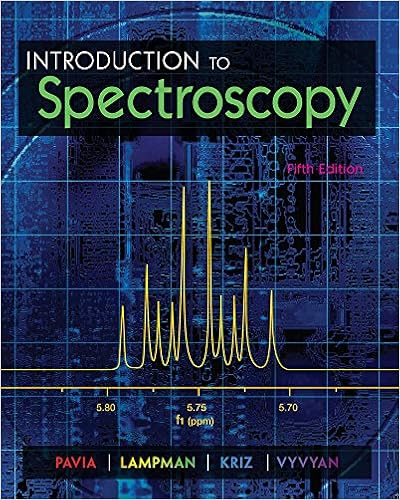# HW8_Ch15_ANS.pdf - Homework 8 Chapter 15 1 At what angle is...

• Homework Help
• achin1004
• 2
• 100% (14) 14 out of 14 people found this document helpful

This preview shows page 1 - 2 out of 2 pages.

HW8_Ch15_ANS.pdf

1 .   At what angle is the n =1 diffraction peak observed when a crystal with d = 1.5 Å is imaged with a Cu atom X- ray source ( λ= 1.54 Å ) ?
a. 19 º
b.
c. 34 º
d. 62
2 .   The resolution limit is a measure of the
3 .   Which method of solving the phase problem involves collecting diffraction patterns for the same crystal at multiple wavelengths to probe core electrons in a protein crystal ?
4 .   Which of the following is NOT true about X-ray diffraction and crystallography ?
5 .   Which of the following is true ?
a .
b . Crystallographic measurements are only possible at synchrotron sources
c . X- rays have energies much smaller than visible light
d . Phases to solve new structures can only be obtained from known homologous proteins
Answer:  a .   Structure factors have amplitude and phase information
6 .   Why are X- rays used for crystallography , compared with other frequencies ?
7 .   Why is small molecule crystallography often simpler than protein or other macromolecule crystallography ?
8 .   Suppose that an EXAFS experiment shows the presence of one peak at 2.3 Å for a protein with a metal cluster . What is an interpretation of this peak ?
9 .   Synchrotrons are useful to
a.
b. assist in crystallization
c. calculate Fourier transforms
d . determine crystal sizes
Answer:  a.   generate brilliant x - rays
10 .   What is NOT true , nitrogenase
11 .   What is NOT true , protein crystals
12 .   Calculate the wavelength associated with the n =3 to n=1 transition in Cu.
13 .   What is the correct order for increasing symmetry in Bravais lattices
a.
b. orthorhombic , triclinic , cubic
c. cubic , triclinic , orthorhombic
d . cubic , orthorhombic , triclinic
Answer:  a.   triclinic , orthorhombic , cubic
14 .   Protein crystallization tests include changing
15 .   Which of the following techniques uses X- rays
##### We have textbook solutions for you!
The document you are viewing contains questions related to this textbook.The document you are viewing contains questions related to this textbook.
Chapter 11 / Exercise 42
Introduction to Spectroscopy
Lampman/PaviaExpert Verified
Homework 8 Chapter 151. At what angle is the n=1 diffraction peak observed when a crystal withd=1.5 Å is imaged with a Cu atom X-raysource ( λ=1.54Å)?a. 19ºb. 31ºc. 34ºd. 62ºsin࠵?=࠵?࠵?2࠵?=(1)(1.54Å)2(1.)=0.513࠵?=31°
2. The resolution limit is a measure of the
3. Which method of solving the phase problem involves collecting diffraction patterns for the same crystal atmultiple wavelengths to probe core electrons in a protein crystal?
4.Which of the following is NOT true about X-ray diffraction and crystallography?
##### We have textbook solutions for you!
The document you are viewing contains questions related to this textbook.The document you are viewing contains questions related to this textbook.
Chapter 11 / Exercise 42
Introduction to Spectroscopy
Lampman/PaviaExpert Verified
•••